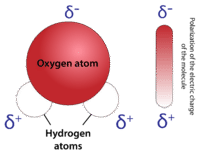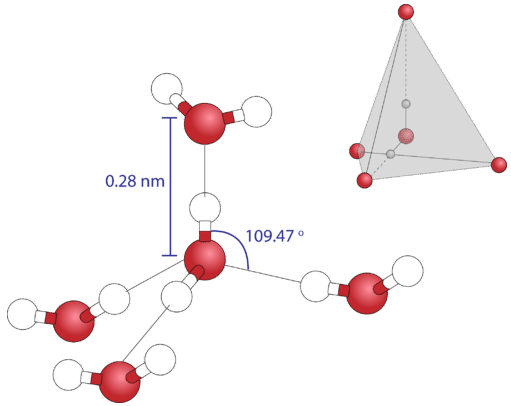# 15.1: Structure of Water

$$\newcommand{\vecs}{\overset { \rightharpoonup} {\mathbf{#1}} }$$ $$\newcommand{\vecd}{\overset{-\!-\!\rightharpoonup}{\vphantom{a}\smash {#1}}}$$$$\newcommand{\id}{\mathrm{id}}$$ $$\newcommand{\Span}{\mathrm{span}}$$ $$\newcommand{\kernel}{\mathrm{null}\,}$$ $$\newcommand{\range}{\mathrm{range}\,}$$ $$\newcommand{\RealPart}{\mathrm{Re}}$$ $$\newcommand{\ImaginaryPart}{\mathrm{Im}}$$ $$\newcommand{\Argument}{\mathrm{Arg}}$$ $$\newcommand{\norm}{\| #1 \|}$$ $$\newcommand{\inner}{\langle #1, #2 \rangle}$$ $$\newcommand{\Span}{\mathrm{span}}$$ $$\newcommand{\id}{\mathrm{id}}$$ $$\newcommand{\Span}{\mathrm{span}}$$ $$\newcommand{\kernel}{\mathrm{null}\,}$$ $$\newcommand{\range}{\mathrm{range}\,}$$ $$\newcommand{\RealPart}{\mathrm{Re}}$$ $$\newcommand{\ImaginaryPart}{\mathrm{Im}}$$ $$\newcommand{\Argument}{\mathrm{Arg}}$$ $$\newcommand{\norm}{\| #1 \|}$$ $$\newcommand{\inner}{\langle #1, #2 \rangle}$$ $$\newcommand{\Span}{\mathrm{span}}$$$$\newcommand{\AA}{\unicode[.8,0]{x212B}}$$

In his well-known poem "The Rime of the Ancient Mariner", Samuel Coleridge wrote "water, water everywhere, nor any drop to drink." Coleridge was talking about being out on the ocean, but not having any water because he had killed an albatross (apparently bringing bad luck to everyone on the ship). About $$75\%$$ of the Earth's surface is water. The major constituent of the human body (over $$60\%$$) is water. This simple molecule plays important roles in all kinds of processes.

## Structure of Water

Water is a simple molecule consisting of one oxygen atom bonded to two different hydrogen atoms. Because of the higher electronegativity of the oxygen atom, the bonds are polar covalent (polar bonds). The oxygen atom attracts the shared electrons of the covalent bonds to a significantly greater extent than the hydrogen atoms. As a result, the oxygen atom acquires a partial negative charge $$\left( \delta - \right)$$, while the hydrogen atoms each acquire a partial positive charge $$\left( \delta + \right)$$. The molecule adopts a bent structure because of the two lone pairs of electrons on the oxygen atom. The $$\ce{H-O-H}$$ bond angle is about $$105^\text{o}$$, slightly smaller than the ideal $$109.5^\text{o}$$ of an $$sp^3$$ hybridized atomic orbital.Figure $$\PageIndex{1}$$: The water molecule, visualized three different ways: ball-and-stick model, space-filling model, and structural formula with partial charges.

The bent shape of the water molecule is critical because the polar $$\ce{O-H}$$ bonds do not cancel one another and the molecule as a whole is polar. The figure below illustrates the net polarity of the water molecule. The oxygen is the negative end of the molecule, while the area between the hydrogen atoms is the positive end of the molecule.Figure $$\PageIndex{2}$$: Water is a polar molecule, as greater electron density is found around the more electronegative oxygen atom.

Polar molecules attract one another by dipole-dipole forces, as the positive end of one molecule is attracted to the negative end of the nearby molecule. In the case of water, the highly polar $$\ce{O-H}$$ bonds results in very little electron density around the hydrogen atoms. Each hydrogen atom is strongly attracted to the lone-pair electrons on an adjacent oxygen atom. These are called hydrogen bonds and are stronger than conventional dipole-dipole forces.Figure $$\PageIndex{3}$$: A hydrogen bond is the attraction between a lone pair of electrons on the oxygen atom of one molecule and the electron-deficient hydrogen atom of a nearby molecule.

Because each oxygen atom has two lone pairs, it can make hydrogen bonds to the hydrogen atoms of two separate other molecules. The figure below shows the result—an approximately tetrahedral geometry around each oxygen atom, consisting of two covalent bonds and two hydrogen bonds.Figure $$\PageIndex{4}$$: As a result of two covalent bonds and two hydrogen bonds, the geometry around each oxygen atom is approximately tetrahedral.

## Summary

• Water is a molecular compound consisting of polar molecules that have a bent shape.
• The oxygen atom acquires a partial negative charge, while the hydrogen atom acquires a partial positive charge.

This page titled 15.1: Structure of Water is shared under a CK-12 license and was authored, remixed, and/or curated by CK-12 Foundation via source content that was edited to the style and standards of the LibreTexts platform; a detailed edit history is available upon request.